A bag contains 8 red, 6 white and 4 black balls. A ball is drawn at random from the bag.
Question:

A bag contains 8 red, 6 white and 4 black balls. A ball is drawn at random from the bag. Find the probability that the drawn ball is

(i) red or white

(ii) not black

(iii) neither white nor black.

Solution:

GIVEN: A bag contains 8 red, 4 black and 6 white balls and a ball is drawn at random

TO FIND: Probability of getting a

(i) red or white ball

(ii) not black ball

(iii) neither white nor black

Total number of balls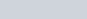(i) Total number red and white balls are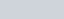We know that PROBABILITY =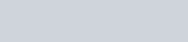Hence probability of getting red or white ball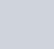(ii) Total number of black balls is 4

We know that PROBABILITY =Hence probability of getting black ball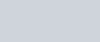We know that sum of probability of occurrence of an event and probability of non occurrence of an event is 1.

$P(\mathrm{E})+P(\overline{\mathrm{E}})=1$

$\frac{2}{9}+\mathrm{P}(\overline{\mathrm{E}})=1$

$\mathrm{P}(\overline{\mathrm{E}})=1-\frac{2}{9}$

$P(\bar{E})=\frac{9-2}{9}$

$P(\bar{E})=\frac{7}{9}$

Hence the probability of getting non black ball is $P(\bar{E})=\frac{7}{9}$

(iii) Total number of neither red nor black balls i.e. red ball is 8

We know that PROBABILITY =Hence probability of getting neither white nor black ball